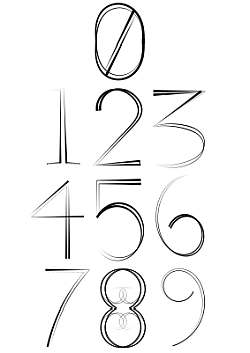# Greatest Common Divisor Calculator

Instructions: Use this Greatest Common Divisor Calculator to compute the Greatest Common Divisor (GCD) for two non-negative integer values $$n_1$$ and $$n_2$$. The values of $$n_1$$ and $$n_2$$ need to be integer and greater than or equal to 1The integer $$n_1$$ =The integer $$n_2$$ =

## How to Compute the Greatest Common Divisor

More about the Greatest Common Divisor (sometimes also referred as Greatest Common Factor) : The greatest common divisor (GCD) between two positive integer numbers $$n_1$$ and $$n_2$$ is the largest integer that divides both $$n_1$$ and $$n_2$$.

The greatest common divisor is broadly used in the manipulation of fraction, especially when we want to reduce fractions, by finding the largest value by which we can divide the numerator and denominator (in other words, the GCD of the numerator and denominator)

Tightly related to the GCD, you have the least common multiple for two numbers a and b, which represents the smallest number that is a multiple of both numbers a and b

## Why do you need to calculate the greatest common divisor?

The greatest common divisor (or also known as GCD) has many uses, one them being for fraction simplification. That is not the only one, for sure. There are a myriad of uses of it in Arithmetics.## How to compute the greatest common divisor?

It is usually easy to find by inspection (this is, trying lots of numbers in a systematic way, until we find it), but that is true only for small numbers.

Computing the GCD for large numbers by inspection can be tedious or plain hard. That is some systematic approach is needed, though in many cases it will be simply very computationally intensive.

Fortunately, there is a systematic, easy (cough, cough) way to compute the GCD for two numbers. The method goes like this

• Step 1: Compute the prime decomposition of $$n_1$$ and $$n_2$$. Symbolically, we would have something like this: $n_1 = p_1^{\alpha_1}\cdot p_2^{\alpha_2}\cdots p_n^{\alpha_n}$ $n_2 = q_1^{\alpha_1}\cdot p_2^{\alpha_2}\cdots p_m^{\alpha_m}$
• Step 2: Find the list of common primes in the corresponding prime decomposition. If there are no common primes, then STOP, you have found that GCD = 1. Otherwise, let $$\{r_1, ..., r_k \}$$ be the list of $$k$$ common primes and let $$\alpha_{i_l}, \beta_{i_l}$$ for $$l=1,2,..,k$$ the corresponding exponents found in the prime decomposition of $$n_1$$ and $$n_2$$ for the corresponding common primes.

• Step 3: The GCD is computed as: $GCD = r_1^{\min\{\alpha_{i_1}, \beta_{i_1}\}} \cdot r_2^{ \min\{\alpha_{i_2}, \beta_{i_2}\}} \cdots r_k^{\min\{\alpha_{i_k}, \beta_{i_k}\}}$## Examples of the calculation of the GCD

The above method looks too complex?? Not really. Let's see an example: Let us compute the GCD for $$n_1 = 165$$ and $$n_2 = 1575$$. Let us find the prime decomposition of each of these numbers (you can use our prime decomposition calculator)

$165 = 3 \cdot 5 \cdot 11$ $1575 = 3^2 \cdot 5^2 \cdot 7$

From the above: what primes do these two numbers have in common? As we can see, the common primes are 3 and 5. Looking at the exponents of these common primes in each of the numbers, we look the minimum between the two. In this case, the minimum exponent for 3 is 1, and the minimum exponent for 5 is also 1. Therefore

$GCD = 3^1 \cdot 5^1 = 3 \cdot 5 = 15$

Aside from the GDC calculator, you can choose among our selection of algebra calculators and solvers .

## Example: GCD calculation

Calculate the greatest common divisor for 12 and 18.

Solution: In this case, the numbers are small enough to do it by inspection. Let's go seeing numbers: 2 divides both 12 and 18. Then 3 also divides 12 and 18.

The number 4 divides 12 but does not divide 18. The number 5 does not divide either of them. Number 6, on the other hand divides both 12 and 18.

Now, any number between 7 and 11 does not divide 12, and 12 does not divide 18.

Conclusion: The greatest common divisor (GCD) of 12 and 18 is 6.

## Other number calculators

Computing the GCD and LCM for two given numbers are tasks of great utility when it comes down to the simplification of algebraic expressions.

Also, it plays a role when dealing with fraction and finding a common denominator, among many others.